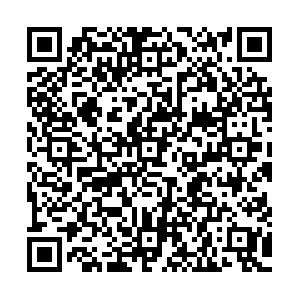# Charmonia and bottomonia in asymmetric magnetized hot nuclear matter

• We investigate the mass-shift of P-wave charmonium (${\chi_c}_0$, ${\chi_c}_1$), and S and P-wave bottomonium ($\eta_b$, $\Upsilon$, ${\chi_b}_0$, and ${\chi_b}_1$) states in magnetized hot asymmetric nuclear matter using the unification of QCD sum rules (QCDSR) and the chiral $SU(3)$model. Within QCDSR, we use two approaches, i.e., the moment sum rule and the Borel sum rule. The magnetic field induced scalar gluon condensate $\left\langle \frac{\alpha_{s}}{\pi} G^a_{\mu\nu} {G^a}^{\mu\nu} \right\rangle$and the twist-2 gluon operator $\left\langle \frac{\alpha_{s}}{\pi} G^a_{\mu\sigma} {{G^a}_\nu}^{\sigma} \right\rangle$calculated in the chiral $SU(3$) model are utilised in QCD sum rules to calculate the in-medium mass-shift of the above mesons. The attractive mass-shift of these mesons is observed, which is more sensitive to magnetic field in the high density regime for charmonium, however less so for bottomonium. These results may be helpful to understand the decay of higher quarkonium states to the lower quarkonium states in asymmetric heavy ion collision experiments.
••Get Citation
. doi: 10.1088/1674-1137/43/12/124109
.Milestone
Revised: 2019-09-26
Article Metric

Article Views(100)
Cited by(0)
Policy on re-use
To reuse of subscription content published by CPC, the users need to request permission from CPC, unless the content was published under an Open Access license which automatically permits that type of reuse.
###### 通讯作者: 陈斌, bchen63@163.com
• 1.

沈阳化工大学材料科学与工程学院 沈阳 110142

Title:
Email:

## Charmonia and bottomonia in asymmetric magnetized hot nuclear matter

###### Corresponding author: Arvind Kumar, iitd.arvind@gmail.com
• Department of Physics, Dr. B R Ambedkar National Institute of Technology Jalandhar, Jalandhar 144011, Punjab, India

Abstract: We investigate the mass-shift of P-wave charmonium (${\chi_c}_0$, ${\chi_c}_1$), and S and P-wave bottomonium ($\eta_b$, $\Upsilon$, ${\chi_b}_0$, and ${\chi_b}_1$) states in magnetized hot asymmetric nuclear matter using the unification of QCD sum rules (QCDSR) and the chiral $SU(3)$model. Within QCDSR, we use two approaches, i.e., the moment sum rule and the Borel sum rule. The magnetic field induced scalar gluon condensate $\left\langle \frac{\alpha_{s}}{\pi} G^a_{\mu\nu} {G^a}^{\mu\nu} \right\rangle$and the twist-2 gluon operator $\left\langle \frac{\alpha_{s}}{\pi} G^a_{\mu\sigma} {{G^a}_\nu}^{\sigma} \right\rangle$calculated in the chiral $SU(3$) model are utilised in QCD sum rules to calculate the in-medium mass-shift of the above mesons. The attractive mass-shift of these mesons is observed, which is more sensitive to magnetic field in the high density regime for charmonium, however less so for bottomonium. These results may be helpful to understand the decay of higher quarkonium states to the lower quarkonium states in asymmetric heavy ion collision experiments.

### HTML关注分享DownLoad:  Full-Size Img  PowerPoint